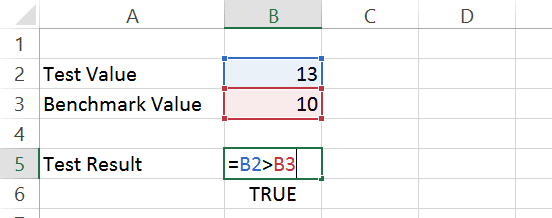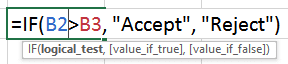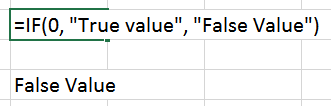# Excel IF function – Dos and Don’tsSo far, I have not written an article about the humble IF function. But in the last few months I came across many customers who asked me to explain how the IF function works. Surprised? The reason is that many people use the Excel IF function, but they do not understand its nuances.

Usually someone has created a file and is handed over from person to person. Worse still, those who know how to use the IF function misuse it! So here it is a simple, short and practical description of how to use the IF function.

## Excel IF function

### The Need

Working with data requires decisions to be taken. Usually decision making has a basis. In terms of Excel, there could be one or more conditions which can lead to a decision. Depending upon whether the condition is met with or not, we want to take different decision. This is where the IF function in Excel is very useful.

### How to check if B2 is greater than the Benchmark?

Let us start with a simple example. We are conducting a test on a product.
We want to know if B2 is greater than the benchmark (B3).

This requires a very simple formula as shown below:= is just to start the formula entry.
Thereafter we are checking if B2 is greater than B3. No Excel function is required.

This formula is like asking Excel a question, “Is B2 greater than B3”? Now, Excel must reply as Yes or No.

Excel is not a human being so it answers in a technical way as TRUE which basically means Yes. If B2 is lesser than or equal to B3, Excel’s answer would be “No” which is shown as FALSE.

If we just wanted to know this information, we have already finished our job.

#### Take an action, based upon the comparison

If B2 is higher than the benchmark, we will accept the product, otherwise reject it because it failed the Quality Check. This is where the IF function is used.Here is another variation.### How does the IF function work?

IF function is just emulating our decision basis. Because it is a function, you must communicate with it by providing relevant data to it.

1. The first parameter is the basis of our decision or the condition.
2. Second parameter is to specify what to do if the condition is TRUE and
3. The third parameter is to say what to do if the condition is FALSE.

While typing the function, this syntax is shown for your reference.Notice that the cursor is positioned inside the comparison. That is the logical_test parameter. Other two parameters are called value_if_true and value_if_false.

That’s it. Practice it a few times and you will get the hang of it.

### What kind of logical test works?

Well, practically any formula will work as a logical test. It should return TRUE or FALSE.

In fact, even non-logical data can also be put as the logical condition.This formula will always return True Value as the result.
Any positive number is considered as logical TRUE. Zero and below is considered as logical FALSE. That is how the Excel IF function works.### More complex decisions using Excel IF function

Let us go back to our Test results example.
Suppose if the test value is above the benchmark, we accept the product.
If it same as or 2 below the benchmark (between 8 and 10) then we must retest.
If it is below benchmark – 2 (less than 8), then we should reject it.#### How does this work?

Look at the Formula syntax below the formula itself.

First IF condition checks if the test value is higher than the benchmark. If TRUE, we Accept.

Now we have one more condition to test – is it higher than 8 or lower than 8. That is being done in the second IF statement. The entire second IF statement is a part of value_if_false part of the first IF statement. Thus, if the first if fails, it tries the second if and returns the desired decision.

### Caution: Too many IFs are usually bad

We saw that with three conditions to check against, we needed two IF statements – one inside the other. What if you had multiple such conditions to check – for example 0 to 2, 2 to 4, 4 to 6 and so on? You will find it very confusing and cumbersome to put IF statements within each other.

We still get the formulas right – somehow. But usually, if there are many such conditions required, VLOOKUP function is a better choice.

Another misuse (and overuse) of related functions like COUNTIF(), SUMIF(), etc. is also a common occurrence. Often, the simpler solution is to use Pivot Tables.## 6 thoughts on “Excel IF function – Dos and Don’ts”

1.Vipul says:

Great Learning

1.Dr Nitin Paranjape says:

There are lot of articles about Excel on my blog. Do explore and let me know your feedback.

2.GAURANG P BHATT says:

I just made an IF statement based calculation. Somehow, I feel it is inefficient. Can you suggest an approach (not solution) to do it more elegantly? The variables are changing so not creating data programmatically as of now.

Product Code Basic Sales rate CGroup1 CGroup 2 CGroup 3
SKU001 100 105 110 115
SKU002 200 210 220 230
SKU004 300 315 330 345
SKU005 400 420 440 460

I can’t attach an Excel sheet nor image here. This is monthly sales rate of product customer group wise. On basis of customer group, certain percentage is applied to basic sale rate.

This is customer group citywise. According to city, it picks up VAT/CST (but that is not through Excel, as program takes care of it.)

CUST001 CG1 Chennai
CUST004 CG3 Mumbai
CUST005 CG2 Jaipur
CUST006 CG1 Bangalore
CUST007 CG3 Pune
CUST008 CG2 Bhopal
CUST009 CG2 Indore
CUST010 CG1 Coimbatore

Sales price is assigned to each customer on the basis of group. So each customer has each SKU price.

I tried cross-tab, but couldn’t achieve the result. So, constructed a nested IF function.

=IF(E17108=\$H\$6,VLOOKUP(B17108,’Sales Price’!\$A\$6:\$P\$423,6,0),IF(E17108=\$I\$6,VLOOKUP(B17108,’Sales Price’!\$A\$6:\$P\$423,8,0),IF(E17108=\$J\$6,VLOOKUP(B17108,’Sales Price’!\$A\$6:\$P\$423,10,0),IF(E17108=\$K\$6,VLOOKUP(B17108,’Sales Price’!\$A\$6:\$P\$423,12,0),IF(E17108=\$L\$6,VLOOKUP(B17108,’Sales Price’!\$A\$6:\$P\$423,14,0),IF(E17108=\$M\$6,VLOOKUP(B17108,’Sales Price’!\$A\$6:\$P\$423,16,0),IF(E17108=\$N\$6,VLOOKUP(B17108,’Sales Price’!\$A\$6:\$P\$423,4,0))))))))

of course, it works, but when a new group will created, a new IF will be added.

final outcome is,

Custcode SKUcode Rate
1 1
2 2
3 3
… …

for each customer.

I save the file as 97-2003 format and convert it to SQL table and import in SQL database. Excel 2016 doesn’t work.

Please guide for an elegant approach.

Gaurang Bhatt

1.Dr Nitin Paranjape says:

Sorry for the delayed reply. The problem is with your SKU table. By adding columns for Customer groups, you already created a cross-tab.
That should be a simple table. The columns should be Product – Code Basic Sales rate – Customer Group – Group Rate. add a combined key containing sKU + CG in the beginning. This will be used for vlookup.
Now a single vlookup with input given as SKU code and customer Group will give you the pricing.